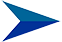The Mandelbrot Fractal
ContentsThe Mandelbrot Fractal Discovered and invented by Benoit MandelbrotInfinitely Complex Infinite complexity in x, y and z (iteration depth) dimensionsInfinite Complexity Proof Formal mathematical proof of infinite complexityGeneralisation of Proof Extending the proof beyond the inner boundary

# The Mandelbrot Fractal

The discoverer and inventor of the Mandelbrot Fractal is Benoit Mandelbrot.

"Mandelbrot ended up doing a great piece of science and identifying a much stronger and more fundamental idea—put simply, that there are some geometric shapes, which he called "fractals", that are equally "rough" at all scales. No matter how close you look, they never get simpler, much as the section of a rocky coastline you can see at your feet looks just as jagged as the stretch you can see from space."

He said of his discovery:

"Exploring this set I certainly never had the feeling of invention. I never had the feeling that my imagination was rich enough to invent all those extraordinary things on discovering them. They were there, even though nobody had seen them before. It's marvelous, a very simple formula explains all these very complicated things. So the goal of science is starting with a mess, and explaining it with a simple formula, a kind of dream of science."

Please take the time to read about him on Wikipedia, he is a true renaissance genius, inventor and computer programmer.

The Mandelbrot Fractal is a truly astonishing mathematical phenomenon, an entire universe that can be explored, as infinite in some respects as the tangible universe. A tall claim? Perhaps, but at some level infinities are equivalent.

Take one of an infinite number of possible journeys into the Mandelbrot Fractal:

The graphic beauty and complexity of the Mandelbrot Fractal has made it famous and has been the focus of many projects.

The underlying numeric complexity is much greater than the colours, as colours are derived with modulus functions that reduce complexity. This underlying numeric complexity has been engineered for Fractal Transformation.

# Infinitely Complex

Exploring the Mandelbrot Fractal graphically (see video link above) you get a sense that it is very, very complex, perhaps even infinitely complex.

Start at a different location, make different turns along the way, and you will take an entirely different journey.

There is no limit to the zoom, it just continues on and on...

When exploring the underlying numerics you notice that adjacent pixels with the same colour actually have very different numeric values.

Even the region usually shown as black, a region where the recursion does not return (infinity), still returns different values for every pixel.

The regions beyond the core 'Mandelbrot Man' continue indefinitely with complex numerics.

It certainly looks like infinite complexity in x, y and z (iteration depth) dimensions.

# Infinite Complexity Proof

Benoit Mandelbrot was the first to theorise that the Mandelbrot Fractal was infinitely complex.

However, there was no formal proof of this until 1991 when mathematical proof was provided by Mitsuhiro Shishikura.

New Scientist Magazine say of this proof that the Mandelbrot Fractal is as complex as it could be.

So the Mandelbrot Fractal must have the greatest possible level of complexity, namely Infinite Complexity.

For the math buffs, here is Mitsuhiro Shishikuras Proof that shows Mandelbrot Fractal has a Hausdorf dimension of 2, being infinitely complex with an infinite distance.

Our understanding of the Mitsuhiro Shishikura proof is that it proves the inner Mandelbrot boundary is an infinitely complex line. The closer you look at it the more convoluted it becomes.

# Generalisation of Proof

Wolfgang Flatow generalises the Mitsuhiro Shishikura proof to include the space surrounding the inner boundary.

It is evident that there is an infinite number of distinct layers, each having a boundary, extending outward from the inner boundary, each with a Hausdorf dimension of 2, being infinitely complex with an infinite distance.

Each layer/border displays a unique shape, different from the previous layer/border.

Each layer/border becomes more convoluted the closer you look at it.

This extends proof of infinite complexity across the entire plane, but for our purposes we restrict the claimed proof to a radius of 4 from the origin.

The Mandelbrot Fractal has a 3rd z dimension in the form of the iteration depths at every conceivable x,y location, an infinite set containing unique values.

The values returned, while consistent, are complex and unpredictable. We therefore generalise the complex boundary proof to include the 3rd z depth dimension and the values returned.